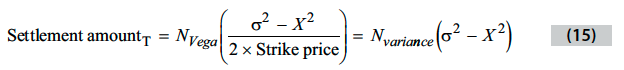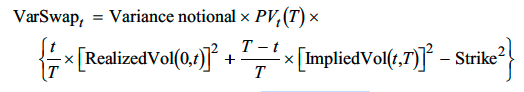# Variance swap: payoff vs gain

Hi guys,
I’m reading variance swap and really couldn’t understand the difference between the two formulas.In the CFA official textbook, these two formulas are for “the payoff of variance swap at time T” and “the value of variance swap at time t”.
But when I was working on practice problems #15 (p127), the answer uses “the value of variance swap at time t” as the gain of the purchase of the variance swap.

Why should we use “the value of variance swap at time t” formula to calculate the gain of the variance swap? I thought that the formula is for the value of variance swap not the gain for the variance swap.

Thanks!

The top formula is for the settlement amount at expiry.

The bottom formula is valuing the var swap at time t (prior to expiry)

How much do you pay to enter into a variance swap?